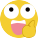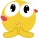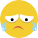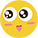# 净利下滑超八成 可口可乐涉足酒饮料市场寻突破

");//加首页首页 else if(countPage>1&¤tPage!=0&¤tPage==1) document.write("上一页"); else document.write(""); //循环 var num = 20; for(var i=0+(currentPage-1-(currentPage-1)%num) ; i<=(num+(currentPage-1-(currentPage-1)%num))&&(i"+(i+1)+""); else{ if(i==0) document.write(""+(i+1)+""); else document.write(""+(i+1)+""); } } //设置下一页代码 if(countPage>1&¤tPage!=(countPage-1)) document.write("下一页");//加尾页尾页 else document.write("");

[责任编辑：糜陆成]

•好文
•钦佩
•喜欢
•泪奔
•可爱
•思考